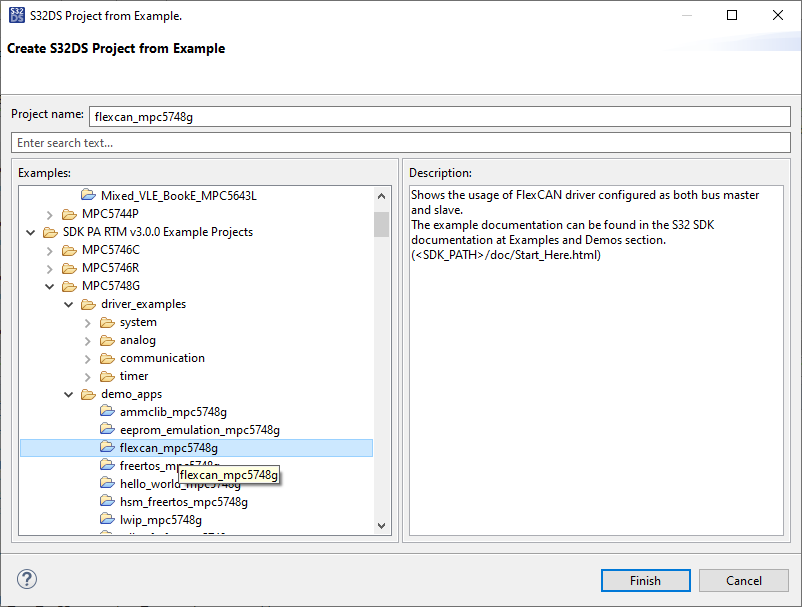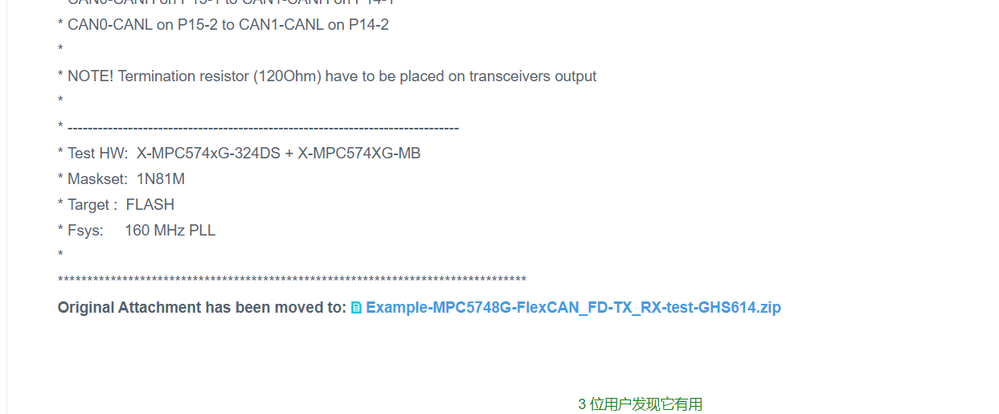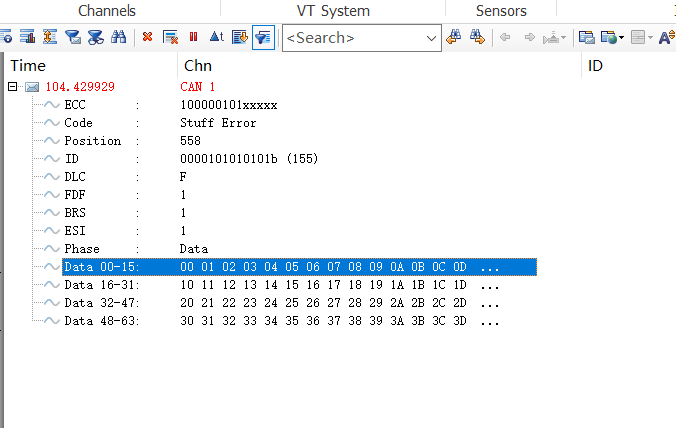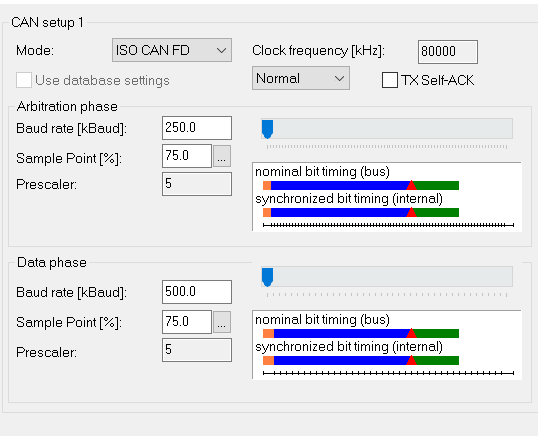## Request a CAN FD Demo for MPC5748G

cancel
Showing results for
Did you mean:
SOLVED

## Request a CAN FD Demo for MPC5748G

388 ViewsContributor III

Hi,

Request a CAN FD Demo for MPC5748G.

Thanks.

Tags (2)
1 Solution
261 ViewsContributor III

Hi Petr,

The problem has been solved. In the upload MPC5748_CAN_FD project,your Flexcan_Init() function  does not set the  CAN_0.CTRL2.R register and enable the  ISO_CAN_FD_ENABLE.This cause the CAN_FD transmit error.

Thank you.

6 Replies
261 ViewsNXP TechSupport

Hi,

for example here; Example MPC5748G FlexCAN FD simple TX/RX GHS614

There are also SDK example within S32 Design Studio which can be modified for CAN FD easilyBR, Petr

261 ViewsContributor III261 ViewsNXP TechSupport

I have uploaded the file again.

BR, Petr

262 ViewsContributor III

Hi Petr,

The problem has been solved. In the upload MPC5748_CAN_FD project,your Flexcan_Init() function  does not set the  CAN_0.CTRL2.R register and enable the  ISO_CAN_FD_ENABLE.This cause the CAN_FD transmit error.

Thank you.

261 ViewsContributor III

Hi Petr,

I have used your upload MPC5748G project, and find it can't run on my MPC5748G  development board, The Can_Init() can't pass ,  so i changed the interface ,and then it works normally.

But during debug the  project, canoe always report  CAN_FD message error. I check the canoe setup ,and find the can_fd baud rate and the sample point is the same as my project , I don't know what cause this problem.Can you check my following can interface , and give me some advice?

thank you .

The can interface as below:

void FlexCAN0_Init(void)
{
uint32_t i = 0;

/* enable the FlexCAN module, reset and freeze */
CAN_0.MCR.B.MDIS = 1; /* Disable module before selecting clock source*/
CAN_0.CTRL1.B.CLKSRC=0; /* Clock Source = oscillator clock (40 MHz) */
CAN_0.MCR.B.MDIS = 0; /* Enable module for config. (Sets FRZ, HALT)*/

/* double check that we are actually in freeze mode */
while(0 == CAN_0.MCR.B.FRZACK) {};

CAN_0.CBT.R =0x808721A7;        // BTF=1;EPRSDIV = 4;ERJW=7;EPRSEG = 8;EPSEG1=13;EPSEG2=7
// clockSrc =40Mhz; braud = 40/((4+1)*(4+8+13+7)) = 250kHz.
// smp = (1+EPRSEG+EPSEG1+2)/(1+EPRSEG+EPSEG1+2+EPSEG2+1) = (1+8+13+2)/(1+8+13+2+7+1)                                                                    =     24/32 = 75%.

CAN_0.FDCBT.R = 0x004310C3;   // FPRESDIV= 4;FRJW=3;FPROSEG=4;FPSEG1=6;FPSEG2=3
//braud =40/((4+1)*(3+4+6+3)) =500kHz.
//smp = (1+FPROSEG+FPSEG1+1)/(1+FPROSEG+FPSEG1+1+FPSEG2+1) = 12/16 = 75%.

CAN_0.FDCTRL.R = 0x80030500;    // TDCEN = 0;TDCOFF = 5;

/* Make first 64 message buffers inactive by writing their control fields
* to "not active". They will be left inactive until we're ready for communication. */
for(i=0;i<96;i++)
{
CAN_0.MB[i].CS.R = 0;
}

/*CAN0 MB寄存器的设置，其中MB设置为发，MB设置为收。*/
// CAN_0.MB.CS.B.IDE = 0; /* MB 0 will look for a standard ID */
// CAN_0.MB.ID.B.ID_STD = 0x155; /* MB 0 will look for ID = 0x555 */
// CAN_0.MB.CS.B.CODE = 4; /* MB 0 set to RX EMPTY */

CAN_0.MB.CS.B.CODE = 8; //MB设置为发送MB

/* configure CAN0TX and CAN0RX pin functions */
SIUL2.MSCR.B.SSS = 1; /* Pad PB0: Source signal is CAN0_TX */
SIUL2.MSCR.B.OBE = 1; /* Pad PB0: Output Buffer Enable */
SIUL2.MSCR.B.SRC = 3; /* Pad PB0: Maximum slew rate */
SIUL2.MSCR.B.IBE = 1; /* Pad PB1: Enable pad for input - CAN0_RX */
SIUL2.IMCR.B.SSS = 2; /* CAN0_RX: connected to pad PB1 */

/* Finally clear the HALT flag in MCR to enable the FlexCAN
* to synchronize with the CAN bus and allow
* participation in communication. */

CAN_0.MCR.R = (0
// | CAN_MCR_BCC /* individual Rx masking and queue */
| CAN_MCR_AEN /* Safe Tx abort enable */
| CAN_MCR_FDEN /* CAN FD enabled */
| 0x0000003F); /* enable 64 MBs */

/* wait until FlexCAN ready */
while(1 == CAN_0.MCR.B.FRZACK){};
while(1 == CAN_0.MCR.B.NOTRDY){};
}

the transmit message as blew：

void TransmitMsgFD(uint8_t ext, uint32_t ID, uint8_t length)
{
/* Assumption: Message buffer CODE is INACTIVE */
uint8_t i,dlc,z=0;
uint32_t *pTxMB = (uint32_t *)&CAN_0.MB.CS.R;
uint32_t txID;

if (ext==0) txID = ID<<18;
else txID = ID;

/*calculate dlc value from no. bytes*/
if(length < 0x09)
{
dlc = length;
}else if(length < 0x0D){
dlc = 0x9;
}else if(length < 0x11){
dlc = 0xA;
}else if(length < 0x15){
dlc = 0xB;
}else if(length < 0x19){
dlc = 0xC;
}else if(length < 0x21){
dlc = 0xD;
}else if(length < 0x31){
dlc = 0xE;
}else{
dlc = 0xF;
}

// *(pTxMB + 1) = txID; // write ID

for (i=0; i<length/4; i++,z+=4) {
*(pTxMB + 2 + i) = (z<<24) | ((z+1)<<16) | ((z+2)<< 8) | (z+3); /* Data to be transmitted */
}

// *pTxMB = (0 // Tx Buffer 0 T0 word
// | 1<<31 // extended Data Length
// | 1<<30 // bit rate switch
// | 0xC<<24 // code = 0xC, TX once
// | 1<<22 // SRR = 1
// | ext<<21 // IDE
// | 0<<20 // RTR = 0
// | dlc<<16); // DLC

CAN_0.MB.CS.B.IDE = 0; /* Use standard ID length */
CAN_0.MB.ID.B.ID_STD = ID; /* Transmit ID is 0x555 */
CAN_0.MB.CS.B.RTR = 0; /* Data frame, not remote Tx request frame */
CAN_0.MB.CS.B.DLC = dlc;

CAN_0.MB.CS.B.EDL = 1;

CAN_0.MB.CS.B.BRS = 1;

CAN_0.MB.CS.B.SRR = 1; /* Tx frame (not req'd for standard frame)*/
CAN_0.MB.CS.B.CODE =0xC; /* Activate msg. buf. to transmit data frame */

}

The can message error and the can_fd set up as blew:the can-fd set up as blew: